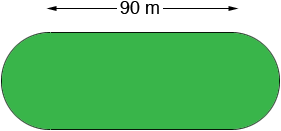SEARCH HOMEMath Central Quandaries & QueriesQuestion from tammy, a student: The inner boundary ABCDEF of an athletic track consists of two straight parts each 90m long and two semi circular ends as shown in the diagram.If the perimeter of the inner boundary is 400m, calculate the diameter AE of the inner semi circle. Part 2. If the track is 3.5m wide an athlete starts the 400m run at X and remains in the outer lane . He finishes at Y(1) Calculate the outer diameter YZ (2)The distance XY (take pie as 22/7)Tammy,

You didn't include a diagram so I drew my own.The straight sides are 90 m each and the total perimeter is 400 m so the semicircular ends have a total perimeter of 400 - (2 × 90) = 220 m. Thus if you put the two semicircles together you have a circle of circumference 220 metres. What is the diameter of a circle with a circumference of 200 metres?

Without the diagram I can't help with part 2 but hopefully what I suggested on part 1 will allow you to complete part 2.

PennyMath Central is supported by the University of Regina and The Pacific Institute for the Mathematical Sciences.